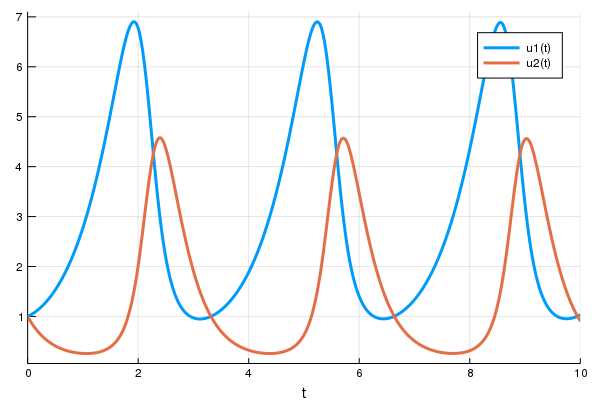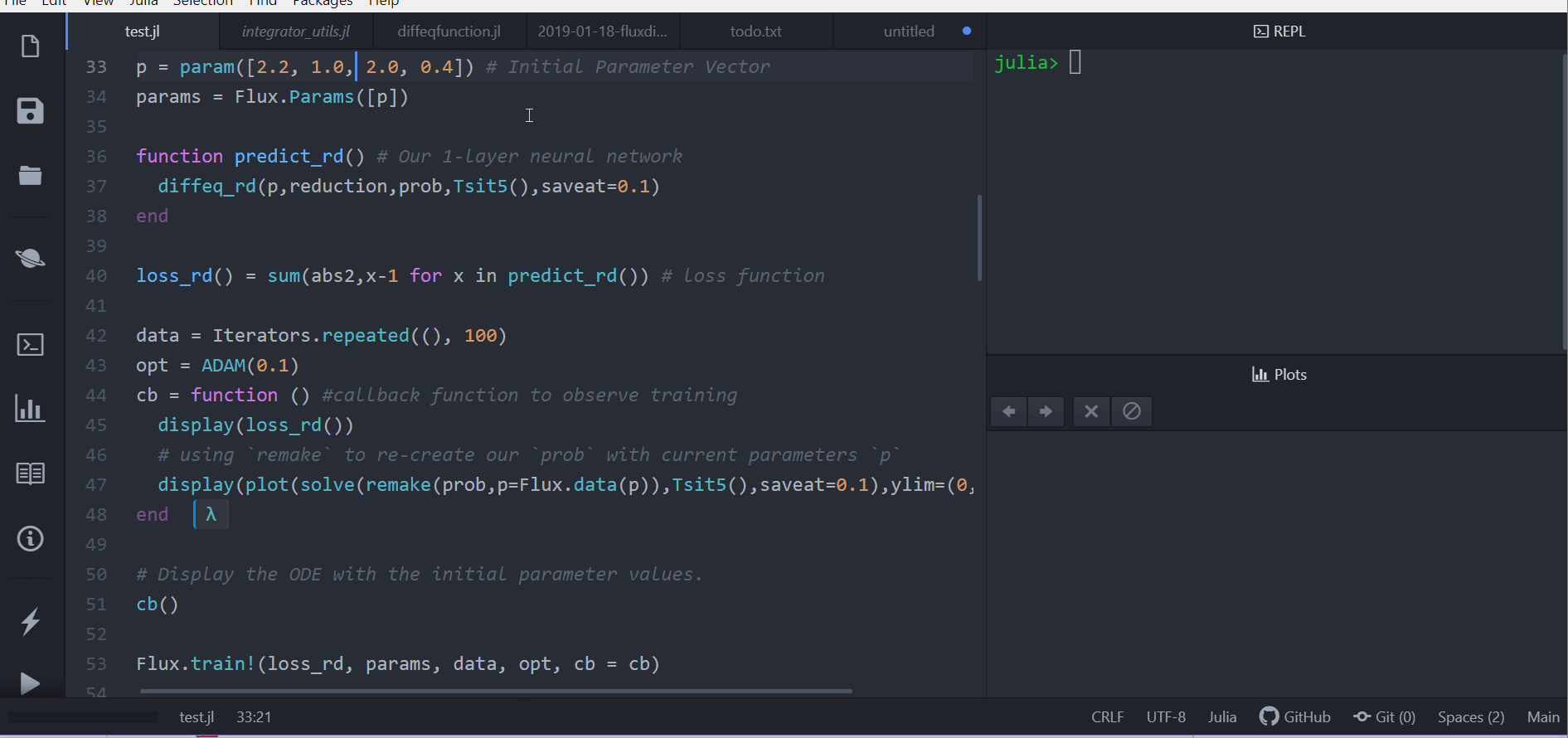# Optimization of Ordinary Differential Equations

## Copy-Paste Code

If you want to just get things running, try the following! Explanation will follow.

using DifferentialEquations, DiffEqFlux, Plots

function lotka_volterra!(du, u, p, t)
x, y = u
α, β, δ, γ = p
du = dx = α*x - β*x*y
du = dy = -δ*y + γ*x*y
end

# Initial condition
u0 = [1.0, 1.0]

# Simulation interval and intermediary points
tspan = (0.0, 10.0)
tsteps = 0.0:0.1:10.0

# LV equation parameter. p = [α, β, δ, γ]
p = [1.5, 1.0, 3.0, 1.0]

# Setup the ODE problem, then solve
prob = ODEProblem(lotka_volterra!, u0, tspan, p)
sol = solve(prob, Tsit5())

# Plot the solution
using Plots
plot(sol)
savefig("LV_ode.png")

function loss(p)
sol = solve(prob, Tsit5(), p=p, saveat = tsteps)
loss = sum(abs2, sol.-1)
return loss, sol
end

callback = function (p, l, pred)
display(l)
plt = plot(pred, ylim = (0, 6))
display(plt)
# Tell sciml_train to not halt the optimization. If return true, then
# optimization stops.
return false
end

result_ode = DiffEqFlux.sciml_train(loss, p,
cb = callback,
maxiters = 100)
# result_ode = DiffEqFlux.sciml_train(loss, p, ADAM(0.1), cb = callback)

## Explanation

First let's create a Lotka-Volterra ODE using DifferentialEquations.jl. For more details, see the DifferentialEquations.jl documentation. The Lotka-Volterra equations have the form:

\begin{aligned} \frac{dx}{dt} &= \alpha x - \beta x y \\ \frac{dy}{dt} &= -\delta y + \gamma x y \\ \end{aligned}
using DifferentialEquations, DiffEqFlux, Plots

function lotka_volterra!(du, u, p, t)
x, y = u
α, β, δ, γ = p
du = dx = α*x - β*x*y
du = dy = -δ*y + γ*x*y
end

# Initial condition
u0 = [1.0, 1.0]

# Simulation interval and intermediary points
tspan = (0.0, 10.0)
tsteps = 0.0:0.1:10.0

# LV equation parameter. p = [α, β, δ, γ]
p = [1.5, 1.0, 3.0, 1.0]

# Setup the ODE problem, then solve
prob = ODEProblem(lotka_volterra!, u0, tspan, p)
sol = solve(prob, Tsit5())

# Plot the solution
using Plots
plot(sol)
savefig("LV_ode.png")For this first example, we do not yet include a neural network. We take AD-compatible solve function function that takes the parameters and an initial condition and returns the solution of the differential equation. Next we choose a loss function. Our goal will be to find parameters that make the Lotka-Volterra solution constant x(t)=1, so we define our loss as the squared distance from 1. Note that when using sciml_train, the first return is the loss value, and the other returns are sent to the callback for monitoring convergence.

function loss(p)
sol = solve(prob, Tsit5(), p=p, saveat = tsteps)
loss = sum(abs2, sol.-1)
return loss, sol
end

Lastly, we use the sciml_train function to train the parameters using ADAM to arrive at parameters which optimize for our goal. sciml_train allows defining a callback that will be called at each step of our training loop. It takes in the current parameter vector and the returns of the last call to the loss function. We will display the current loss and make a plot of the current situation:

callback = function (p, l, pred)
display(l)
plt = plot(pred, ylim = (0, 6))
display(plt)
# Tell sciml_train to not halt the optimization. If return true, then
# optimization stops.
return false
end

Let's optimize the model.

result_ode = DiffEqFlux.sciml_train(loss, p, cb = callback)

In just seconds we found parameters which give a relative loss of 1e-16! We can get the final loss with result_ode.minimum, and get the optimal parameters with result_ode.u. For example, we can plot the final outcome and show that we solved the control problem and successfully found parameters to make the ODE solution constant:

remade_solution = solve(remake(prob, p = result_ode.u), Tsit5(),
saveat = tsteps)
plot(remade_solution, ylim = (0, 6))Note that this was done with the default optimizer. One can also pass an optimization method, like ADAM(0.1), and tweak settings like set maxiters=100 to force at most 100 iterations of the optimization. This looks like:

result_ode = DiffEqFlux.sciml_train(loss, p, ADAM(0.1), cb = callback, maxiters=100)